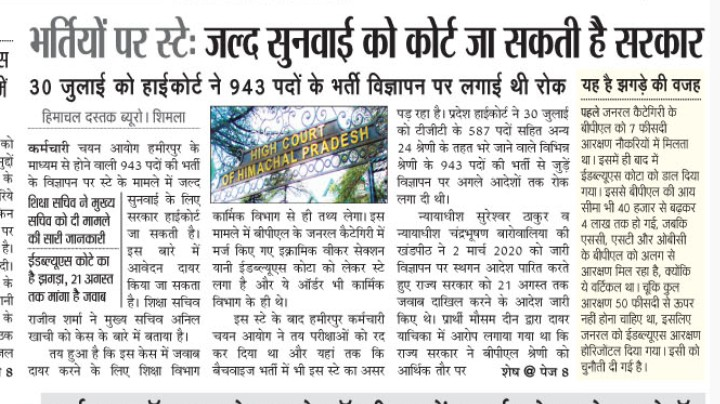### 3 साल बाद 1156 पदों पर भर्ती का नतीजा, 560 पदों पर नहीं मिले कोई योग्य उम्मीदवार

HPPSC TOADY DECLARES THE FINAL RESULT FOR 1156,POSTS. In continuation to the Final result declared on 23.02.2019 for filling up
1156 posts {General(UR)=525, General(BPL)=109, General(WFF)=15, OBC(UR)=170,
OBC(BPL)=38, OBC(WFF)=03, SC(UR)=207, SC(BPL)=35, SC(WFF)=07, ST(UR)=35,
ST(BPL)=11& ST(WFF)=01} of Jr. Office Assistant (on regular & contract basis) Post
Code: 556, which were received from various departments of Himachal Pradesh and were
th October, 2016. Out of 1156 post 596
posts were filled up and 560 posts {General(UR)=170, General(BPL)=78, General(WFF)=14,
OBC(UR)=105, OBC(BPL)=31, OBC(WFF)=03, SC(UR)=111, SC(BPL)=09, SC(WFF)=07,
ST(UR)=28, ST(BPL)=03 & ST(WFF)=01} remained vacant due to non availability of suitable
candidates. Further out of 560 vacant posts 08 posts of General (UR) and 02 posts of SC(UR)
categories were kept vacant due to the Hon’ble HP Administrative Tribunal directions in OA
No. 2644/2019.